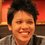# Chebyshev Polynomials Proof Problem

Prove (or disprove): Define $T_n(x)$ as the Chebyshev polynomial of the first kind with degree $n$. If $p$ is an odd prime, then $\sqrt{\frac{T_p(x) - 1}{x-1}}$ is an irreducible polynomials over the rational numbers.

By definition $T_n(x) = \cos(n \cos^{-1}(x))$. Listing out the first few odd primes of $n$ shows that (for simplicity sake, we take the positive root only)

$T_3(x) - 1 = (4x^3-3x) - 1 = (x-1)(2x+1)^2 \\ \Rightarrow \sqrt{\frac{T_3(x) - 1}{x-1}} = 2x + 1$

$T_5(x) - 1 = (16x^5-20x^3+5x) - 1 = (x-1)(4x^2+2x-1)^2 \\ \Rightarrow \sqrt{\frac{T_5(x) - 1}{x-1}} = 4x^2+2x-1$

$T_7(x) - 1 = (64x^7-112x^5+56x^3-7x) - 1 = (x-1)(8x^3+4x^2-4x-1)^2 \\ \Rightarrow \sqrt{\frac{T_7(x) - 1}{x-1}} = 8x^3+4x^2-4x-1$

\begin{aligned} T_{11}(x) - 1 &=& (1024 x^{11}-2816 x^9+2816 x^7-1232 x^5+220 x^3-11x) - 1 \\ &=& (x-1) (32 x^5+16 x^4-32 x^3-12 x^2+6 x+1)^2 \\ \Rightarrow \sqrt{\frac{T_{11}(x) - 1}{x-1}} &=& 32 x^5+16 x^4-32 x^3-12 x^2+6 x+1 \end{aligned}

All these polynomials are irreducible polynomials over the rationals. Is it true for all odd prime $p$?Note by Pi Han Goh
6 years, 1 month ago

This discussion board is a place to discuss our Daily Challenges and the math and science related to those challenges. Explanations are more than just a solution — they should explain the steps and thinking strategies that you used to obtain the solution. Comments should further the discussion of math and science.

When posting on Brilliant:

• Use the emojis to react to an explanation, whether you're congratulating a job well done , or just really confused .
• Ask specific questions about the challenge or the steps in somebody's explanation. Well-posed questions can add a lot to the discussion, but posting "I don't understand!" doesn't help anyone.
• Try to contribute something new to the discussion, whether it is an extension, generalization or other idea related to the challenge.

MarkdownAppears as
*italics* or _italics_ italics
**bold** or __bold__ bold
- bulleted- list
• bulleted
• list
1. numbered2. list
1. numbered
2. list
Note: you must add a full line of space before and after lists for them to show up correctly
paragraph 1paragraph 2

paragraph 1

paragraph 2

[example link](https://brilliant.org)example link
> This is a quote
This is a quote
    # I indented these lines
# 4 spaces, and now they show
# up as a code block.

print "hello world"
# I indented these lines
# 4 spaces, and now they show
# up as a code block.

print "hello world"
MathAppears as
Remember to wrap math in $$ ... $$ or $ ... $ to ensure proper formatting.
2 \times 3 $2 \times 3$
2^{34} $2^{34}$
a_{i-1} $a_{i-1}$
\frac{2}{3} $\frac{2}{3}$
\sqrt{2} $\sqrt{2}$
\sum_{i=1}^3 $\sum_{i=1}^3$
\sin \theta $\sin \theta$
\boxed{123} $\boxed{123}$

Sort by:

The solution is as I expected click here.I hope you don't mind that I copied the problem to math overflow.It uses only the multiplicative property of the degrees of number fields and that $x^{p-1}+x^{p-2}+...+1$, the pth cyclotomic polynomial, is irreducible (can be proven by shifting x to x+1 and using eisenstein).

- 6 years, 1 month ago

Haha, it's a bit of an overkill to use OverFlow! I was about to post this in StackExchange if there's no answer. Thanks!

- 6 years, 1 month ago

I posted it on stackexchange, but no one provided a good solution :D

- 6 years, 1 month ago

If you shift x to x+1 the irreducibility of these can be proven with Eisenstein's criterion.

- 6 years, 1 month ago

Thanks! But how do I prove that it's true for ALL odd primes $p$?

- 6 years, 1 month ago

By the way do you have a solution or just spotted that they are irreducible for small values?Vecause I can't figure out what identity to use to make that square root in a better form.Eisenstein still works though

- 6 years, 1 month ago

I don't have a solution, hence the "or disprove" statement. I got inspired by solving via the Challenge Master notes comment on my solution here.

- 6 years, 1 month ago

So it is perfectly possible that a counterexample exists.Well I'm going to read up on Tschebyscheff Polynomials, any reccomendations?Also, I have found a paper that states that (T_p(x)/x/) is irreducible for all primes p.But I don't know how to deal with that -1.

- 6 years, 1 month ago

Recommendation? Haha, no, I read up a lot before posting this, and I'm still pretty stumped. Link please?

- 6 years, 1 month ago

I think that the identity $T_n(x)=cos(n.arccosx)$ can give us the roots of $T_n(x)-1$

- 6 years, 1 month ago

- 6 years, 1 month ago

Well cosx is 1 only when x is 2.pi.something.So that means that $n.arrcosx$ must be 2.pi.something.So $x=cos(2.\pi.k/n)$ are the roots of T_n(x)-1.Problem is, I don't know when they double roots and how to prove that T is their minimal polynomial.It is interesting that these roots are the real parts of the roots of unity.

- 6 years, 1 month ago

Also the identity $(T_n(x)-1)/(x-1)(T_n(x)+1)/(x+1)=U_{n-1}$ might help, since we know the factorisation of U.

- 6 years, 1 month ago

This post needs to be seen by more people so we can actually solve it lol.Also I still cannot understand why Eisenstein works here (what is special about the coeffs)

- 6 years, 1 month ago